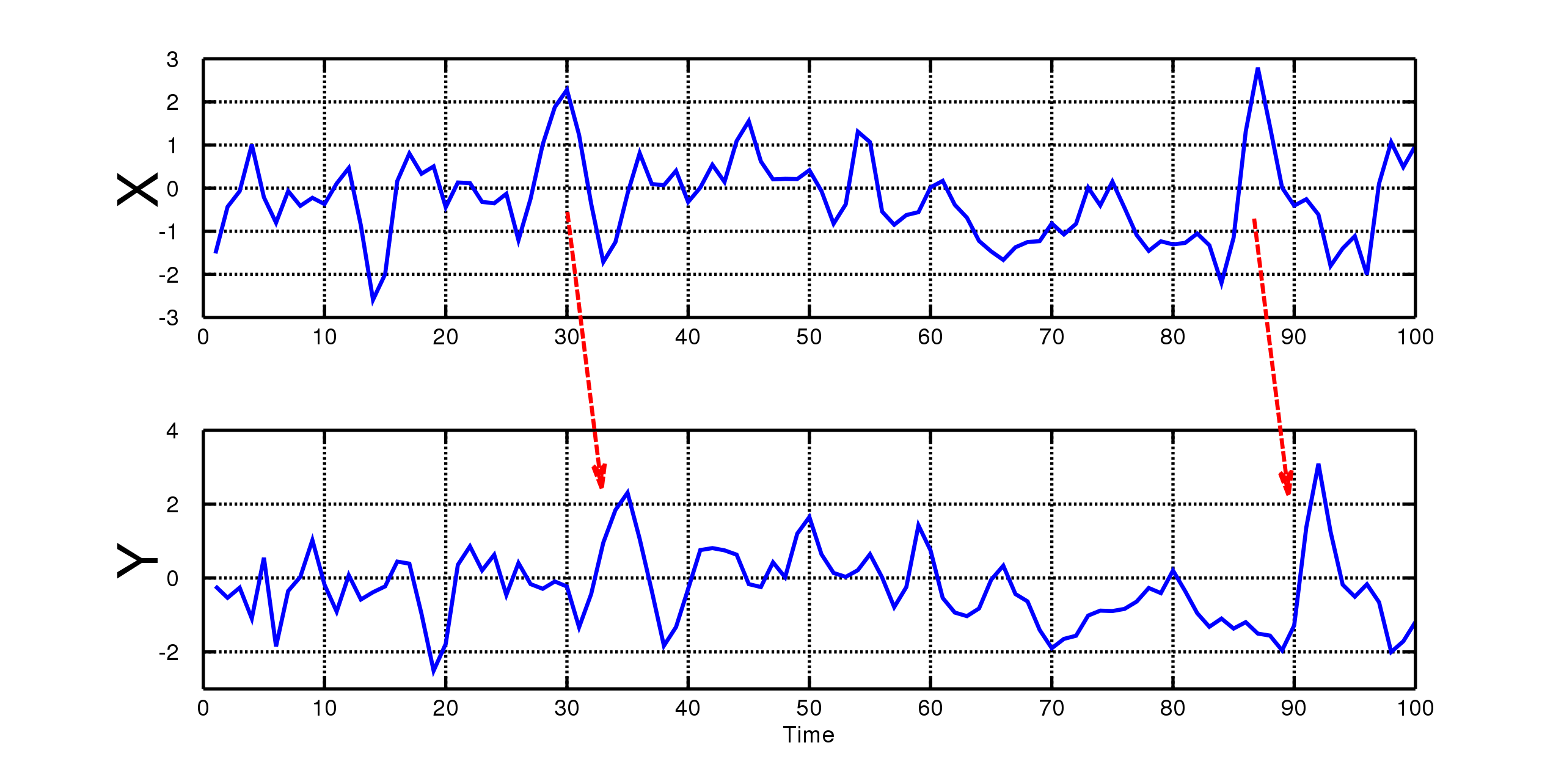Choose the act that is most effective in bringing about the best result. Use causal conditional probabilities instead of evidential probabilities to compute expected utility.

When states are causally independent of the actions (e.g., when they are fixed prior to the choice), use Dominance Reasoning (see DUI).

## Subjunctive Conditionals §

means that if I were to do X, then Y.

• is the causal conditional probability of Y given that I do X
• To calculate, fix the causal history of the world up to the moment you do or don’t do X
• Then determine how your choice of X or not X influences the probability of Y
• Normally, this is equivalent to but not true for Newcomb’s Problem
• is the evidential conditional probability of Y given X

## Causal Dependence §

Three cases

1. Act A causes of influences state S
2. State S causes or influences act A
3. Some common C causes or influences both S and A

Note some properties:

• In all cases,
• In cases 2 and 3 in other words, A has no effect on S

## Granger Causality §

The Granger causality test is a statistical hypothesis test for determining whether one time series is useful in forecasting another, first proposed in 1969.

A time series X is said to Granger-cause Y if it can be shown, usually through a series of t-tests and F-tests on lagged values of X (and with lagged values of Y also included), that those X values provide statistically significant information about future values of Y.Using the term “causality” alone is a misnomer, as Granger-causality is better described as “precedence”, or, as Granger himself later claimed in 1977, “temporally related”. Rather than testing whether X causes Y, the Granger causality tests whether X forecasts Y.